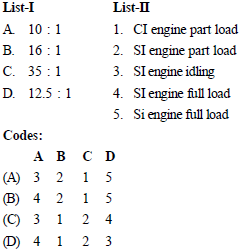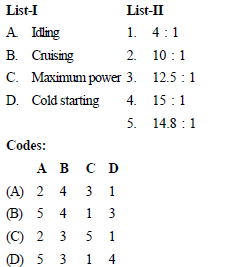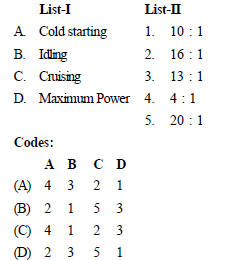# Test: IC Engine Level - 2

## 25 Questions MCQ Test Mechanical Engineering SSC JE (Technical) | Test: IC Engine Level - 2

Description
Attempt Test: IC Engine Level - 2 | 25 questions in 50 minutes | Mock test for Mechanical Engineering preparation | Free important questions MCQ to study Mechanical Engineering SSC JE (Technical) for Mechanical Engineering Exam | Download free PDF with solutions
QUESTION: 1

Solution:
QUESTION: 2

Solution:
QUESTION: 3

### The amount of CO2 produced by 1 kg of carbon on complete combustion is

Solution:
QUESTION: 4

Methane burns with stoichiometric quantity of air. The air-fuel ratio by weight is

Solution:
QUESTION: 5

Match List-I (Air-fuel ratio by mass) with List- II (Engine operation mode) and select correct answer using the codes given below the lists:Solution:
QUESTION: 6

Stoichiometric air-fuel ratio by volume for combustion of methane in air is :

Solution:
QUESTION: 7

Auto- ignition time for petrol-air mixture is minimum when the ratio of actual fuel-air ratio and chemically correct fuel-air ratio is

Solution:
QUESTION: 8

In spark ignition engines knocking can be reduced by:

Solution:
QUESTION: 9

The tendency of knocking in CI engine reduced by

Solution:
QUESTION: 10

Match List-I (SI engine Operational Mode) with List-II (Air Fuel Ratio by Mass) and select correct answer using the codes given below the lists:Solution:
QUESTION: 11

Match List-I (SI engine Aperational Mode) with List-II (Opproximate A/F Ratio) and select correct answer using the codes given below the lists:Solution:
QUESTION: 12

The purpose of employing supercharging for an engine is

Solution:
QUESTION: 13

What is the main objective of supercharging of the engine?

Solution:
QUESTION: 14

For minimizing knocking tendency in SI engine, where should the spark plug be located?

Solution:
QUESTION: 15

What is the purpose of supercharging an engine?

Solution:
QUESTION: 16

Increase in the compression ratio in Otto cycle may cause

Solution:
QUESTION: 17

Pick the odd one

Solution:
QUESTION: 18

Most high speed compression engines operate on

Solution:

Most high speed compression engines operate on Dual combustion cycle

QUESTION: 19

The intake charge in a diesel engine consists of

Solution:
QUESTION: 20

This is considered as the first link in transmitting the gas forces to the output shaft

Solution:
QUESTION: 21

The purpose of balance weight in crankshaft is to

Solution:
QUESTION: 22

Consider the following parts of IC Engine :
1. Rocker arm 2. Follower
3. Cams 4. Camshaft
5. Crankshaft
Which among the above constitutes the part of valve train system ?

Solution:
QUESTION: 23

Gudgeon pin forms the link between

Solution:
QUESTION: 24

Engine of different cylinder dimensions, power and speed are compared on the basis of

Solution:
QUESTION: 25

All of the following are correct except : Inlet-valve mach index is dependent on

Solution:Use Code STAYHOME200 and get INR 200 additional OFF Use Coupon Code